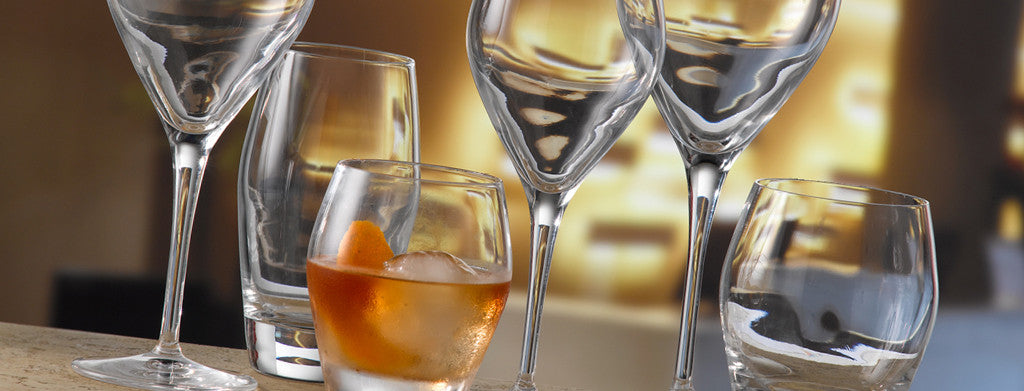Shopping Cart

### Refine

View all

#### Price# Glassware£4.64 each (ex VAT)£3.57 each (ex VAT)£3.44 each (ex VAT)£7.21 each (ex VAT)£6.16 each (ex VAT)£45.03 each (ex VAT)£12.97 each (ex VAT)£8.41 each (ex VAT)£8.08 each (ex VAT)£3.56 each (ex VAT)£3.89 each (ex VAT)£2.87 each (ex VAT)£5.68 each (ex VAT)£11.37 each (ex VAT)£23.14 each (ex VAT)£19.81 each (ex VAT)£19.66 each (ex VAT)£15.31 each (ex VAT)£23.39 each (ex VAT)£10.83 each (ex VAT)£7.43 each (ex VAT)£7.10 each (ex VAT)£10.50 each (ex VAT)£4.00 each (ex VAT)£2.85 each (ex VAT)£6.10 each (ex VAT)£11.58 each (ex VAT)£11.67 each (ex VAT)£15.59 each (ex VAT)£10.41 each (ex VAT)£11.85 each (ex VAT)£3.33 each (ex VAT)£4.52 each (ex VAT)£7.52 each (ex VAT)£4.19 each (ex VAT)£3.00 each (ex VAT)£7.19 each (ex VAT)£3.16 each (ex VAT)£5.14 each (ex VAT)£3.49 each (ex VAT)£2.11 each (ex VAT)£3.77 each (ex VAT)£21.65 each (ex VAT)£3.56 each (ex VAT)£3.23 each (ex VAT)£5.53 each (ex VAT)£6.78 each (ex VAT)£8.43 each (ex VAT)